BoxRatios

Plot3D, SurfaceGraphics and any functions that return SurfaceGraphics have the BoxRatios option with the default setting {1,1,0.4}. When this default setting is used the length of the two horizontal axes is always equal, and the height of the vertical axis is 40% of either horizontal axis. Using this default setting in the next line one gets the impression that the function rapidly oscillates.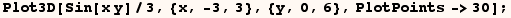Instead one can set BoxRatios to Automatic to ensure that the resulting graphic is not distorted in that each axis is displayed using the same scale.  When the previous line is evaluated with BoxRatios set to Automatic, the figure is relatively short in the vertical direction since the values of Sin[x y]/3 varies over [-1/3, 1/3] which is much less than the variation of  x, y values . You might find that the line below gives a more accurate impression of how rapidly the function oscillates.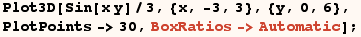Obviously setting BoxRatios to Automatic would be a very bad choice to plot (Sin[x y]/100) over the same plot range as in the last example.

Contrary to the way Plot3D works ParametricPlot3D sets BoxRatios to Automatic by default.  This default setting is used in the next example and produces a nice parametric plot of a 3D-spiral.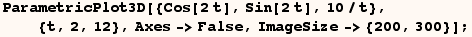Now in the next line the default setting for BoxRatios produces a graph that's very tall and thin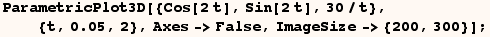A better presentation of the last graphic is made by setting BoxRatios to {1,1,0.4} in the next line.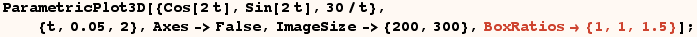Graphics3D also set BoxRatios to Automatic by default.  In addition the following functions in the standard packages set BoxRatios to Automatic by default;
ListPlotVectorField3D
PlotVectorField3D
PlotGradientField3D
SphericalPlot3D
CylindricalPlot3D
PointParametricPlot3D
ListSurfacePlot3D
ScatterPlot3D
ContourPlot3D
ListContourPlot3D

I know of no other functions with the default setting  (BoxRatios→Automatic).

Created by Mathematica  (May 16, 2004)

Back to Ted’s Tricks index page